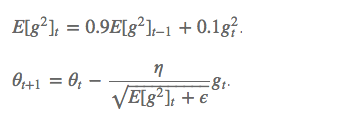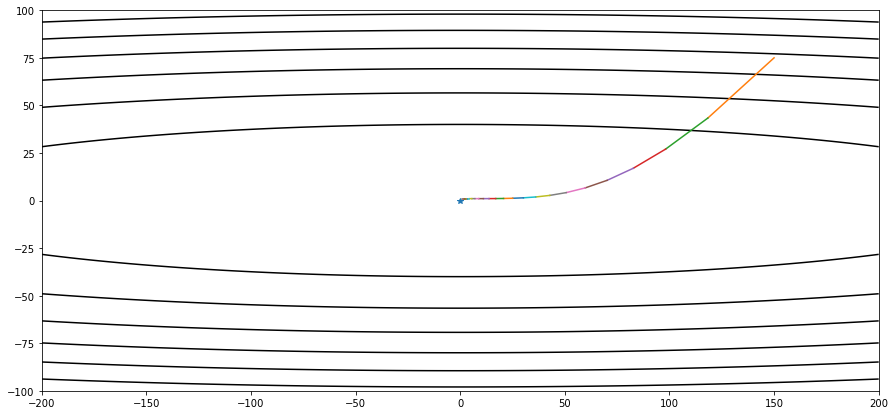RMSProp算法

算法内容

之前说过指数加权移动平均。不同于AdaGrad算法里状态变量 s t \boldsymbol{s}_t 是截至时间步 t t 所有小批量随机梯度 g t \boldsymbol{g}_t 按元素平方和，RMSProp算法将这些梯度按元素平方做指数加权移动平均

具体来说，给定超参数 0 ≤ γ < 1 0 \leq \gamma < 1 ，RMSProp算法在时间步 t > 0 t>0 计算

s t ← γ s t − 1 + ( 1 − γ ) g t ⊙ g t . \boldsymbol{s}_t \leftarrow \gamma \boldsymbol{s}_{t-1} + (1 - \gamma) \boldsymbol{g}_t \odot \boldsymbol{g}_t.

x t ← x t − 1 − η s t + ϵ ⊙ g t , \boldsymbol{x}_t \leftarrow \boldsymbol{x}_{t-1} - \frac{\eta}{\sqrt{\boldsymbol{s}_t + \epsilon}} \odot \boldsymbol{g}_t,

其中

• η \eta 是学习率
• ϵ \epsilon 是为了维持数值稳定性而添加的常数，如 1 0 − 6 10^{-6}

因为RMSProp算法的状态变量 s t \boldsymbol{s}_t 是对平方项 g t ⊙ g t \boldsymbol{g}_t \odot \boldsymbol{g}_t 的指数加权移动平均，所以可以看作是最近 1 / ( 1 − γ ) 1/(1-\gamma) 个时间步的小批量随机梯度平方项的加权平均。如此一来，自变量每个元素的学习率在迭代过程中就不再一直降低（或不变）。

还是使用相同的例子

• 目标函数 f ( x ) = 0.1 x 1 2 + 2 x 2 2 f(\boldsymbol{x})=0.1x_1^2+2x_2^2
• 学习率为0.4

from matplotlib import pyplot as plt

def show_trace_2d(f, results):
plt.plot(*zip(*results), '-o', color='#ff7f0e')
x1, x2 = np.meshgrid(np.arange(-5.5, 1.0, 0.1), np.arange(-3.0, 1.0, 0.1))
plt.contour(x1, x2, f(x1, x2), colors='#1f77b4')
plt.xlabel('x1')
plt.ylabel('x2')

def train_2d(trainer):
x1, x2, s1, s2 = -5, -2, 0, 0  # s1和s2是自变量状态，本章后续几节会使用
results = [(x1, x2)]
for i in range(20):
x1, x2, s1, s2 = trainer(x1, x2, s1, s2)
results.append((x1, x2))
print('epoch %d, x1 %f, x2 %f' % (i + 1, x1, x2))
return results

%matplotlib inline
import math
import torch

def rmsprop_2d(x1, x2, s1, s2):
g1, g2, eps = 0.2 * x1, 4 * x2, 1e-6
s1 = gamma * s1 + (1 - gamma) * g1 ** 2
s2 = gamma * s2 + (1 - gamma) * g2 ** 2
x1 -= eta / math.sqrt(s1 + eps) * g1
x2 -= eta / math.sqrt(s2 + eps) * g2
return x1, x2, s1, s2

def f_2d(x1, x2):
return 0.1 * x1 ** 2 + 2 * x2 ** 2

eta, gamma = 0.4, 0.9
show_trace_2d(f_2d, train_2d(rmsprop_2d))实现RMSProp算法

def get_data_ch7():
data = np.genfromtxt('data/airfoil_self_noise.dat', delimiter='\t')
data = (data - data.mean(axis=0)) / data.std(axis=0)
torch.tensor(data[:1500, -1], dtype=torch.float32) # 前1500个样本(每个样本5个特征)

features, labels = get_data_ch7()

def init_rmsprop_states():
s_w = torch.zeros((features.shape, 1), dtype=torch.float32)
s_b = torch.zeros(1, dtype=torch.float32)
return (s_w, s_b)

def rmsprop(params, states, hyperparams):
gamma, eps = hyperparams['gamma'], 1e-6
for p, s in zip(params, states):
s.data = gamma * s.data + (1 - gamma) * (p.grad.data)**2
p.data -= hyperparams['lr'] * p.grad.data / torch.sqrt(s + eps)

将初始学习率设为0.01，并将超参数 γ \gamma 设为0.9。此时，变量 s t \boldsymbol{s}_t 可看作是最近 1 / ( 1 − 0.9 ) = 10 1/(1-0.9) = 10 个时间步的平方项 g t ⊙ g t \boldsymbol{g}_t \odot \boldsymbol{g}_t 的加权平均。

def train_ch7(optimizer_fn, states, hyperparams, features, labels,
batch_size=10, num_epochs=2):
# 初始化模型
net, loss = linreg, squared_loss

w = torch.nn.Parameter(torch.tensor(np.random.normal(0, 0.01, size=(features.shape, 1)), dtype=torch.float32),

def eval_loss():
return loss(net(features, w, b), labels).mean().item()

ls = [eval_loss()]
torch.utils.data.TensorDataset(features, labels), batch_size, shuffle=True)

for _ in range(num_epochs):
start = time.time()
for batch_i, (X, y) in enumerate(data_iter):
l = loss(net(X, w, b), y).mean()  # 使用平均损失

# 梯度清零

l.backward()
optimizer_fn([w, b], states, hyperparams)  # 迭代模型参数
if (batch_i + 1) * batch_size % 100 == 0:
ls.append(eval_loss())  # 每100个样本记录下当前训练误差
# 打印结果和作图
print('loss: %f, %f sec per epoch' % (ls[-1], time.time() - start))
set_figsize()
plt.plot(np.linspace(0, num_epochs, len(ls)), ls)
plt.xlabel('epoch')
plt.ylabel('loss')

train_ch7(rmsprop, init_rmsprop_states(), {'lr': 0.01, 'gamma': 0.9}, features, labels)亦可以使用pytorch内置的optim.RMSProp算法来实现：

train_pytorch_ch7(torch.optim.RMSprop, {'lr': 0.01, 'alpha': 0.9},
features, labels)

展开全文深度学习 算法
• 深度学习中的RMSprop算法原理

千次阅读 2020-04-08 11:15:30
在https://blog.csdn.net/gaoxueyi551/article/details/105238182一文中，说明了基于Momentum的算法原理，本文介绍的RMSprop算法的引入背景是和Momentum算法相同的，底层理论依然是指数加权平均。 但是，两者优化...

在 https://blog.csdn.net/gaoxueyi551/article/details/105238182 一文中，说明了基于Momentum的算法，本文介绍的RMSprop算法的引入背景和Momentum算法相同，底层理论依然是指数加权平均。

但是，两者优化思路存在些微差别。为了和Momentum算法中的符号相区别，引入向量  ，并设 ，令

其中， 表示对向量的各个分量分别计算平方， 不妨令 ，则得到如下式所示向量，

则  展开也是一个向量，每个分量是权重  对应分量值的平方的指数加权移动平均，

其中，分量值较大的表示权重  对应分量值的平均变化幅度较大，分量值较小的表示权重  对应分量值的平均变化幅度较小。

基于上述定义，给定学习率 ，基于RMSprop的梯度更新规则为，

对于偏置的更新方法类似，不再详述。

Momentum 和 RMSprop算法的共同点如下：

1、减小了梯度在变化幅度较大的分量上的更新速度，提高了变化幅度较小的分量上的更新速度；

2、都对梯度更新的方向进行了优化；

3、长期的优化效果一致，即加快收敛；

4、都使用了指数移动加权平均来对历史梯度信息做处理。

两者的区别如下：

1、前者利用了历史梯度的指数移动加权平均，没有显式的考虑梯度的范数大小，后者是对历史梯度各个分量的二范数进行指数移动加权平均，显式的在梯度各个分量的数值大小上做手脚，在梯度值较大的分量上减小更新步伐，在梯度值较小的分量上增大更新步伐，没有显式的考虑梯度方向

2、从RMSprop公式上看，它可以实现学习率自适应调整，变化较大的梯度分量上的学习率会自动减小，变化较小的梯度分量上的学习率会自动增大。

展开全文神经网络 机器学习 深度学习

千次阅读 2019-12-27 15:21:52

RMSProp算法

RMSProp算法将截止时间 t t 步所有小批量随机梯度 g t g_t 按元素平方做指数加权移动平均。具体来说，给定超参数 0 ≤ γ < 1 0\leq\gamma <1 ,RMSProp算法在时间步 t > 0 t>0 计算
s t ← γ s t − 1 + ( 1 − γ ) g t ⊙ g t s_t\leftarrow\gamma s_{t-1} + (1-\gamma)g_t\odot g_t
然后将损失函数自变量中每个元素的学习率通过按元素运算重新调整，然后更新自变量
x t ← x t − 1 − η s t + ϵ ⊙ g t x_t\leftarrow x_{t-1}- \frac {\eta} {\sqrt {s_t+\epsilon}}\odot g_t
其中 η \eta 是学习率， ϵ \epsilon 是为了维持数值稳定性而添加的常数。因为RMSProp算法的状态变量是对平方项 g t ⊙ g t g_t\odot g_t 的指数加权移动平均，所以可以看作最近 1 / ( 1 − γ ) 1/(1-\gamma) 个时间步的小批量随机梯度平方项的加权平均。如此一来，自变量每个元素的学习率在迭代过程中就不在一直降低（或不变）

AdaDelta算法也像RMSProp算法一样，使用了小批量随机梯度 g t g_t 按元素平方的指数加权移动平均变量 s t s_t 。在时间步0，它的所有元素被初始化为0。给定超参数 0 ≤ ρ < 1 0\leq\rho<1 ，在时间步 t > 0 t>0 ，同RMSProp算法一样计算
s t ← ρ s t − 1 + ( 1 − ρ ) g t ⊙ g t s_t\leftarrow\rho s_{t-1} + (1-\rho)g_t\odot g_t
与RMSProp算法不同的是，AdaDelta算法还维护一个额外的状态变量 Δ x t \Delta x_t ，其元素同样在时间步0被初始化为0。使用 Δ x t − 1 \Delta x_{t-1} 来计算自变量的变化量：
g t ′ = Δ x t − 1 + ϵ s t + ϵ ⊙ g t g_t^{'} = \sqrt {\frac {\Delta x_{t-1} + \epsilon } { s_t + \epsilon}}\odot g_t ϵ \epsilon 是为了维持数值稳定性而添加的常数
接着跟新自变量：
x t ← x t − 1 − g t ′ x_t\leftarrow x_{t-1}-g_t^{'}
最后，我们使用 Δ x t \Delta x_t 来记录自变量变化量 g t ′ g_t{'} 按元素平方的指数加权移动平均：
Δ x t ← ρ Δ x t − 1 + ( 1 − ρ ) g t ′ ⊙ g t ′ \Delta x_t\leftarrow \rho\Delta x_{t-1}+(1-\rho)g_t^{'}\odot g_t^{'}
如不考虑 ϵ \epsilon 的影响，AdaDelta算法与RMSProp算法的不同之处在于使用 Δ x t − 1 \sqrt{\Delta x_{t-1}} 来代替超参数 η \eta

展开全文• Pytorch Note18 优化算法4 RMSprop算法 文章目录Pytorch Note18 优化算法4 RMSprop算法RMSpropRMSProp 算法代码从0实现pytorch内置优化器可视化 全部笔记的汇总贴：Pytorch Note 快乐星球 RMSprop RMSprop 是由 ...

Pytorch Note18 优化算法4 RMSprop算法

全部笔记的汇总贴：Pytorch Note 快乐星球

RMSprop

RMSprop 是由 Geoff Hinton 在他 Coursera 课程中提出的一种适应性学习率方法，至今仍未被公开发表。前面我们提到了 Adagrad 算法有一个问题，就是学习率分母上的变量 s 不断被累加增大，最后会导致学习率除以一个比较大的数之后变得非常小，这不利于我们找到最后的最优解，所以 RMSProp 的提出就是为了解决这个问题。

RMSProp 算法

s i = γ s i − 1 + ( 1 − γ )   g 2 s_i = \gamma s_{i-1} + (1 - \gamma) \ g^2

η s + ϵ \frac{\eta}{\sqrt{s + \epsilon}}

这里 α \alpha 是一个移动平均的系数，也是因为这个系数，导致了 RMSProp 和 Adagrad 不同的地方，这个系数使得 RMSProp 更新到后期累加的梯度平方较小，从而保证 s 不会太大，⾃变量每个元素的学习率在迭代过程中就不再⼀直降低，也就使得模型后期依然能够找到比较优的结果。

简单来说,使用的是指数加权平均，旨在消除梯度下降中的摆动，与Momentum的效果一样，某一维度的导数比较大，则指数加权平均就大，某一维度的导数比较小，则其指数加权平均就小，这样就保证了各维度导数都在一个量级，进而减少了摆动。允许使用一个更大的学习率ηHinton 建议设定γ为 0.9, 学习率 η 为 0.001。

代码从0实现

我们还是利用minst的数据

import numpy as np
import torch
from torchvision.datasets import MNIST # 导入 pytorch 内置的 mnist 数据
from torch import nn
import time
import matplotlib.pyplot as plt
%matplotlib inline

def data_tf(x):
x = np.array(x, dtype='float32') / 255
x = (x - 0.5) / 0.5 # 标准化，这个技巧之后会讲到
x = x.reshape((-1,)) # 拉平
x = torch.from_numpy(x)
return x

# 定义 loss 函数
criterion = nn.CrossEntropyLoss()

def rmsprop(parameters, sqrs, lr, alpha):
eps = 1e-10
for param, sqr in zip(parameters, sqrs):
sqr[:] = alpha * sqr + (1 - alpha) * param.grad.data ** 2
div = lr / torch.sqrt(sqr + eps) * param.grad.data
param.data = param.data - div

# 使用 Sequential 定义 3 层神经网络
net = nn.Sequential(
nn.Linear(784, 200),
nn.ReLU(),
nn.Linear(200, 10),
)

# 初始化梯度平方项
sqrs = []
for param in net.parameters():
sqrs.append(torch.zeros_like(param.data))

# 开始训练
losses = []
idx = 0
start = time.time() # 记时开始
for e in range(5):
train_loss = 0
for im, label in train_data:
im = Variable(im)
label = Variable(label)
# 前向传播
out = net(im)
loss = criterion(out, label)
# 反向传播
loss.backward()
rmsprop(net.parameters(), sqrs, 1e-3, 0.9) # 学习率设为 0.001，alpha 设为 0.9
# 记录误差
train_loss += loss.data
if idx % 30 == 0:
losses.append(loss.data)
idx += 1
print('epoch: {}, Train Loss: {:.6f}'
.format(e, train_loss / len(train_data)))
end = time.time() # 计时结束
print('使用时间: {:.5f} s'.format(end - start))

epoch: 0, Train Loss: 0.381906
epoch: 1, Train Loss: 0.174923
epoch: 2, Train Loss: 0.128508
epoch: 3, Train Loss: 0.104501
epoch: 4, Train Loss: 0.091484
使用时间: 35.81994 s

x_axis = np.linspace(0, 5, len(losses), endpoint=True)
plt.semilogy(x_axis, losses, label='alpha=0.9')
plt.legend(loc='best')我们调整一下我们alpha的值

# 使用 Sequential 定义 3 层神经网络
net = nn.Sequential(
nn.Linear(784, 200),
nn.ReLU(),
nn.Linear(200, 10),
)

# 初始化梯度平方项
sqrs = []
for param in net.parameters():
sqrs.append(torch.zeros_like(param.data))

# 开始训练
losses = []
idx = 0

start = time.time() # 记时开始
for e in range(5):
train_loss = 0
for im, label in train_data:
im = Variable(im)
label = Variable(label)
# 前向传播
out = net(im)
loss = criterion(out, label)
# 反向传播
loss.backward()
rmsprop(net.parameters(), sqrs, 1e-3, 0.999) # 学习率设为 0.001，alpha 设为 0.999
# 记录误差
train_loss += loss.data
if idx % 30 == 0:
losses.append(loss.data)
idx += 1
print('epoch: {}, Train Loss: {:.6f}'
.format(e, train_loss / len(train_data)))
end = time.time() # 计时结束
print('使用时间: {:.5f} s'.format(end - start))

epoch: 0, Train Loss: 0.482363
epoch: 1, Train Loss: 0.169266
epoch: 2, Train Loss: 0.132606
epoch: 3, Train Loss: 0.111919
epoch: 4, Train Loss: 0.096000
使用时间: 32.14718 s

x_axis = np.linspace(0, 5, len(losses), endpoint=True)
plt.semilogy(x_axis, losses, label='alpha=0.999')
plt.legend(loc='best')可以看到使用了不同的 alpha 会使得 loss 在下降过程中的震荡程度不同

pytorch内置优化器

当然 pytorch 也内置了 rmsprop 的方法，非常简单，只需要调用 torch.optim.RMSprop() 就可以了，下面是例子

# 使用 Sequential 定义 3 层神经网络
net = nn.Sequential(
nn.Linear(784, 200),
nn.ReLU(),
nn.Linear(200, 10),
)

optimizer = torch.optim.RMSprop(net.parameters(), lr=1e-3, alpha=0.9)

# 开始训练

start = time.time() # 记时开始
for e in range(5):
train_loss = 0
for im, label in train_data:
im = Variable(im)
label = Variable(label)
# 前向传播
out = net(im)
loss = criterion(out, label)
# 反向传播
loss.backward()
optimizer.step()
# 记录误差
train_loss += loss.data
print('epoch: {}, Train Loss: {:.6f}'
.format(e, train_loss / len(train_data)))
end = time.time() # 计时结束
print('使用时间: {:.5f} s'.format(end - start))

epoch: 0, Train Loss: 0.378526
epoch: 1, Train Loss: 0.167142
epoch: 2, Train Loss: 0.124586
epoch: 3, Train Loss: 0.102094
epoch: 4, Train Loss: 0.088567
使用时间: 37.01984 s

可视化展开全文深度学习 python 神经网络 机器学习 pytorch
• RMSProp算法 RMSProp算法全称root mean square prop算法。 从前面的例子可以知道，当我们利用梯度下降法的时候，虽然横轴也在前进，但纵轴摆动太大。假设纵轴是b横轴是w，假如我们想减弱b的幅度，加快或者不减少...
• 全称是root mean square prop算法 我们假设纵轴方向为b，横轴方向为W。我么希望纵轴slow，横轴fast。 对于第t次迭代，公式如上图。...RMSprop算法一方面能将找到成本函数J最小值的路径从紫色变...
• RMSprop算法全称是root mean square prop算法，该算法可以加速梯度下降，回忆一下之前的例子，如果执行梯度下降，虽然横轴方向正在推进，但纵轴方向会有大幅度的摆动，假设纵轴代表参数b，横轴代表参数W，可能有W1W_...深度学习 优化算法 人工智能 机器学习
• 本文中的这些算法将会对此做出改进。 首先看一下动量法的迭代步骤作为以下各种算法的对比： 设时间步ttt的自变量为xt\boldsymbol{x}_txt​，学习率为ηt\eta_tηt​。 在时间步000，动量法创建速度变量v0\boldsym...随机梯度下降 python 机器学习 深度学习 人工智能
• 假如梯度一直都是g，那么会一直在-g上不停加速，直到达到最终速度，其中步长变为，如当a=0.9时对应最大速度十倍于梯度下降算法。实际中，一开始是一个较小的值。 同时，使用动量以后，会累积之前的梯度，假如梯度...算法 深度学习...